# Digital Electronics - Boolean Algebra and Logic Simplification - Discussion

Discussion Forum : Boolean Algebra and Logic Simplification - General Questions (Q.No. 4)
4.

Derive the Boolean expression for the logic circuit shown below: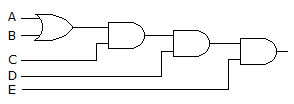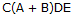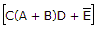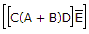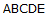Explanation:
No answer description is available. Let's discuss.
Discussion:
9 comments Page 1 of 1.

DAKSHU said:   3 years ago
Thank you all.

Vishnu said:   4 years ago
1+1 = 2.

The binary value of 2 is 10 hence 1+0=1.

Hence proved.

Orwa dalmas said:   5 years ago
Thanks all for explaining.

Veyant said:   6 years ago
Yeah, option 1is correct but explain me the associativity and explain the difference between opt 1&opt c.

Parag said:   8 years ago
1+1=1.

In Boolean algebra its a rule, 1+1=1.

Thanks for you explaination. And any one here please can prove 1+1=1 using boolean algebra expration? Thanks again.

OR GATE OP=(A+B)
1ST AND OP=(A+B)C
2ND AND OP=[(A+B)C]D
3RD AND OP=[(A+B)C]D)E
ANS: [(A+B)C]D)E ....{AFTER SIMPLIFICATION}

Vinay sharma(shoolini university-73) said:   1 decade ago
B and D are not corret because there is bar on E, and in option D it should be A+B because there is or gate for this input. So option A is correct.